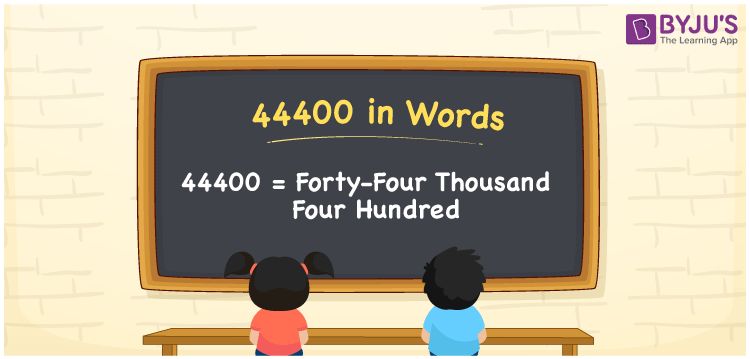# 44400 in Words

44400 in words is Forty-Four Thousand Four Hundred. For instance, if you bought a sofa set worth Rs. 44400, then you can say, “I bought a sofa set worth Rupees Forty-Four Thousand Four Hundred”. Using the place value of 44400, we can convert the number into the word form. Thus, a place value chart is very important to write the number names with ease. 44400 is a cardinal number as it represents a specific amount.

 44400 in Words Forty-Four Thousand Four Hundred Forty-Four Thousand Four Hundred in numerical form 44400

## 44400 in English Words

We generally write numbers in words using the English alphabet. Hence, we can read the number 44400 in English as Forty-Four Thousand Four Hundred.## How to Write 44400 in Words?

The number name of 44400 is written by identifying the place value of the number. The place value chart for the number 44400 is provided below.

 Ten-Thousands Thousands Hundreds Tens Ones 4 4 0 0 0

Therefore, we can write the expanded form as:

4 x Ten Thousand + 4 x Thousand + 4 x Hundred + 0 x Ten + 0 x One

= 4 x 10000 + 4 x 1000 + 4 x 100 + 0 x 10 + 0 x 1

= 40000 + 4000 + 400 + 0 + 0

= 40000 + 4000 + 400

= 44400

= Forty-Four Thousand Four Hundred

Hence, 44400 in words is written as Forty-Four Thousand Four Hundred

Interesting way of writing 44400 in words

4 = Four

44 = Forty-Four

444 = Four Hundred and Forty-Four

4440 = Four Thousand Four Hundred Forty

44400 = Forty-Four Thousand Four Hundred

Thus, the word form of the number 44400 is Forty-Four Thousand Four Hundred

44400 is a natural number that precedes 44401 and succeeds 44399.

• 44400 in words – Forty-Four Thousand Four Hundred
• Is 44400 an odd number? – No
• Is 44400 an even number? – Yes
• Is 44400 a perfect square number? – No
• Is 44400 a perfect cube number? – No
• Is 44400 a prime number? – No
• Is 44400 a composite number? – Yes

## Frequently Asked Questions on 44400 in Words

Q1

### How to write 44400 in words?

44400 in words is written as Forty-Four Thousand Four Hundred.
Q2

### Simplify 40000 + 4400, and express in words.

Simplifying 40000 + 4400, we get 44400. Thus, 44400 in words is Forty-Four Thousand Four Hundred.
Q3

### 44400 is a composite number. True or False.

True, the number 44400 is a composite number.